# Matplotlib的三维可视化，mplot3d教程

2016/01/11 15:48

Matplotlib的三维可视化，mplot3d教程。

# mplot3d tutorial

Contents

## Getting started

An Axes3D object is created just like any other axes using the projection=‘3d’ keyword. Create a new matplotlib.figure.Figure and add a new axes to it of type Axes3D:

import matplotlib.pyplot as plt
from mpl_toolkits.mplot3d import Axes3D

fig = plt.figure()
ax = fig.add_subplot(111, projection='3d')

New in version 1.0.0: This approach is the preferred method of creating a 3D axes.

Note

Prior to version 1.0.0, the method of creating a 3D axes was different. For those using older versions of matplotlib, changeax = fig.add_subplot(111, projection='3d')to ax = Axes3D(fig).

## Line plots

• Axes3D.plot(xs, ys, *args, **kwargs)

• Plot 2D or 3D data.

Argument Description
xs, ys x, y coordinates of vertices
zs z value(s), either one for all points or one for each point.
zdir Which direction to use as z (‘x’, ‘y’ or ‘z’) when plotting a 2D set.

Other arguments are passed on toplot()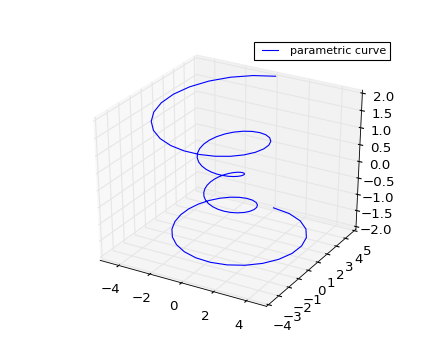## Scatter plots

• Axes3D.scatter(xs, ys, zs=0, zdir='z', s=20, c='b', depthshade=True, *args, **kwargs)

• Create a scatter plot.

Argument Description
xs, ys Positions of data points.
zs Either an array of the same length as xs andys or a single value to place all points in the same plane. Default is 0.
zdir Which direction to use as z (‘x’, ‘y’ or ‘z’) when plotting a 2D set.
s Size in points^2.  It is a scalar or an array of the same length as x and y.
c A color. c can be a single color format string, or a sequence of color specifications of length N, or a sequence of N numbers to be mapped to colors using thecmap and norm specified via kwargs (see below). Note that c should not be a single numeric RGB or RGBA sequence because that is indistinguishable from an array of values to be colormapped.  c can be a 2-D array in which the rows are RGB or RGBA, however.
depthshade Whether or not to shade the scatter markers to give the appearance of depth. Default is True.

Keyword arguments are passed on toscatter().

Returns a Patch3DCollection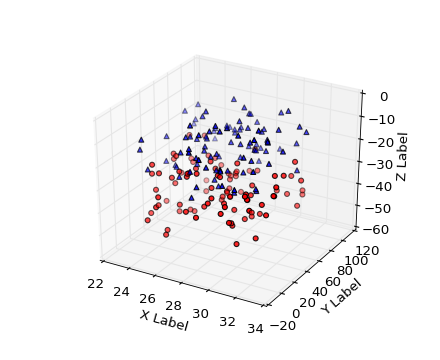## Wireframe plots

• Axes3D.plot_wireframe(X, Y, Z, *args, **kwargs)

• Plot a 3D wireframe.

The rstride and cstride kwargs set the stride used to sample the input data to generate the graph. If either is 0 the input data in not sampled along this direction producing a 3D line plot rather than a wireframe plot.

Argument Description
X, Y, Data values as 2D arrays
Z
rstride Array row stride (step size), defaults to 1
cstride Array column stride (step size), defaults to 1

Keyword arguments are passed on toLineCollection.

Returns a Line3DCollection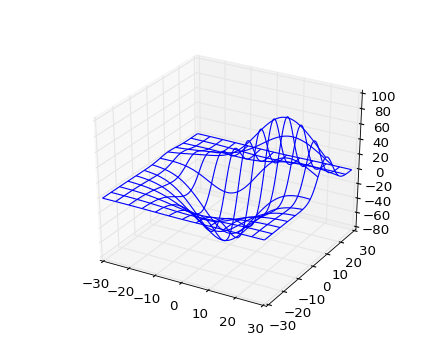## Surface plots

• Axes3D.plot_surface(X, Y, Z, *args, **kwargs)

• Create a surface plot.

By default it will be colored in shades of a solid color, but it also supports color mapping by supplying the cmapargument.

The rstride and cstride kwargs set the stride used to sample the input data to generate the graph.  If 1k by 1k arrays are passed in the default values for the strides will result in a 100x100 grid being plotted.

Argument Description
X, Y, Z Data values as 2D arrays
rstride Array row stride (step size), defaults to 10
cstride Array column stride (step size), defaults to 10
color Color of the surface patches
cmap A colormap for the surface patches.
facecolors Face colors for the individual patches
norm An instance of Normalize to map values to colors
vmin Minimum value to map
vmax Maximum value to map

Other arguments are passed on toPoly3DCollection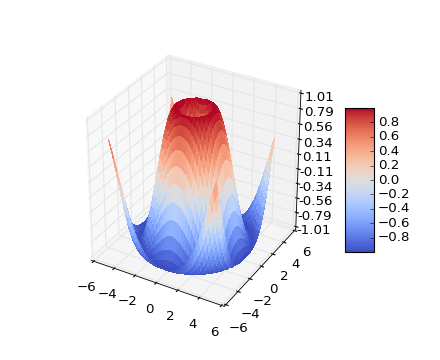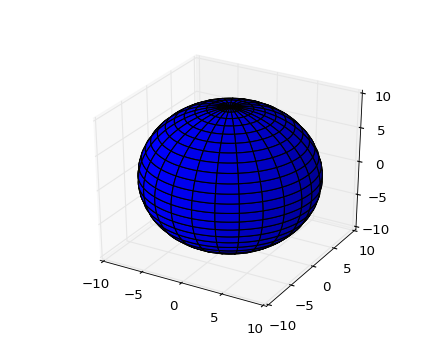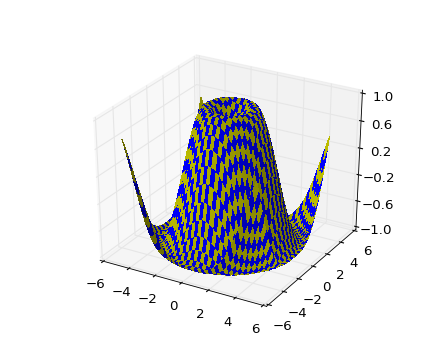## Tri-Surface plots

• Axes3D.plot_trisurf(*args, **kwargs)

• Argument Description
X, Y, Z Data values as 1D arrays
color Color of the surface patches
cmap A colormap for the surface patches.
norm An instance of Normalize to map values to colors
vmin Minimum value to map
vmax Maximum value to map

The (optional) triangulation can be specified in one of two ways; either:

plot_trisurf(triangulation, ...)

where triangulation is a Triangulationobject, or:

plot_trisurf(X, Y, ...)plot_trisurf(X, Y, triangles, ...)plot_trisurf(X, Y, triangles=triangles, ...)

in which case a Triangulation object will be created.  SeeTriangulation for a explanation of these possibilities.

The remaining arguments are:

plot_trisurf(..., Z)

where Z is the array of values to contour, one per point in the triangulation.

Other arguments are passed on toPoly3DCollection

Examples: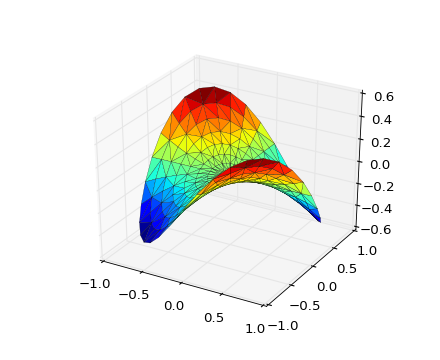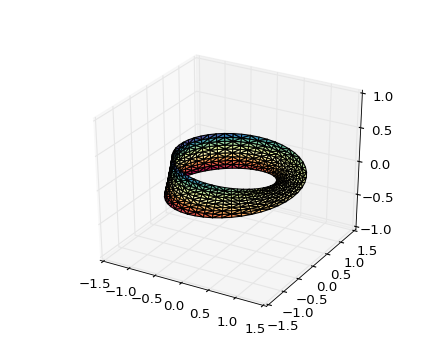(png, hires.png, pdf)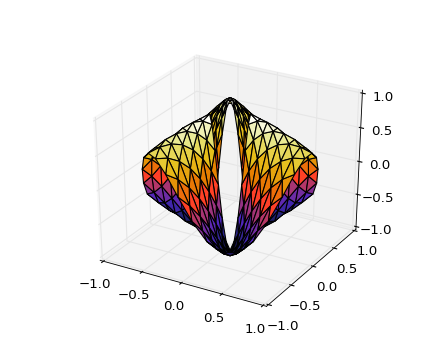(png, hires.png, pdf)

New in version 1.2.0: This plotting function was added for the v1.2.0 release.## Contour plots

• Axes3D.contour(X, Y, Z, *args, **kwargs)

• Create a 3D contour plot.

Argument Description
X, Y, Data values as numpy.arrays
Z
extend3d Whether to extend contour in 3D (default: False)
stride Stride (step size) for extending contour
zdir The direction to use: x, y or z (default)
offset If specified plot a projection of the contour lines on this position in plane normal to zdir

The positional and other keyword arguments are passed on tocontour()

Returns a contour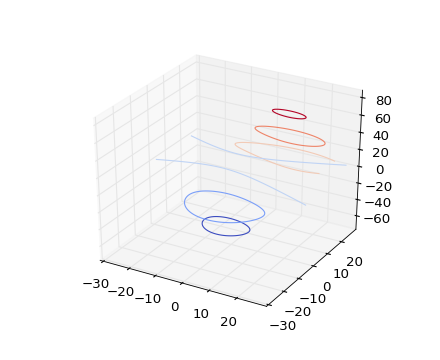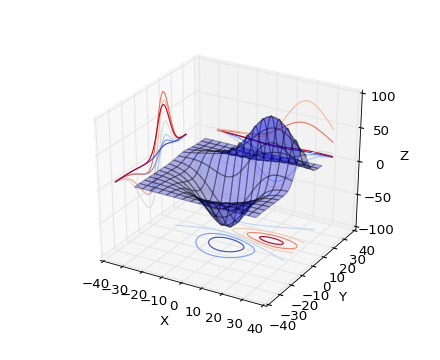## Filled contour plots

• Axes3D.contourf(X, Y, Z, *args, **kwargs)

• Create a 3D contourf plot.

Argument Description
X, Y, Data values as numpy.arrays
Z
zdir The direction to use: x, y or z (default)
offset If specified plot a projection of the filled contour on this position in plane normal to zdir

The positional and keyword arguments are passed on tocontourf()

Returns a contourf

Changed in version 1.1.0: The zdir and offset kwargs were added.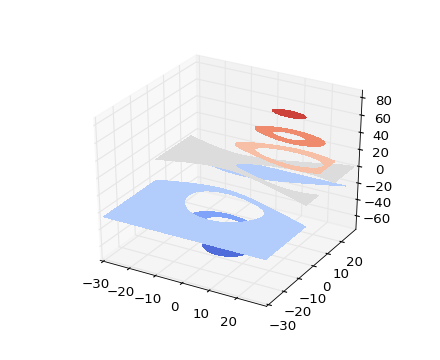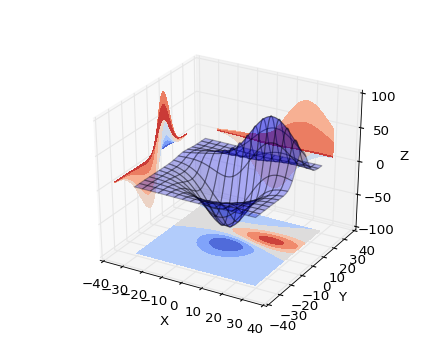New in version 1.1.0: The feature demoed in the second contourf3d example was enabled as a result of a bugfix for version 1.1.0.

## Polygon plots

• Axes3D.add_collection3d(col, zs=0, zdir='z')

• Add a 3D collection object to the plot.

2D collection types are converted to a 3D version by modifying the object and adding z coordinate information.

• PolyCollection

• LineColleciton

• PatchCollection

• Supported are: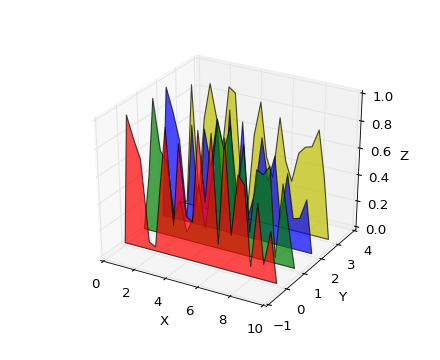## Bar plots

• Axes3D.bar(left, height, zs=0, zdir='z', *args, **kwargs)

Argument Description
left The x coordinates of the left sides of the bars.
height The height of the bars.
zs Z coordinate of bars, if one value is specified they will all be placed at the same z.
zdir Which direction to use as z (‘x’, ‘y’ or ‘z’) when plotting a 2D set.

Keyword arguments are passed onto bar().

Returns a Patch3DCollection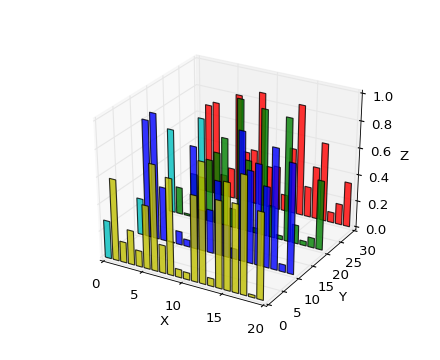## Quiver

• Axes3D.quiver(*args, **kwargs)

• Plot a 3D field of arrows.

call signatures:

quiver(X, Y, Z, U, V, W, **kwargs)

Arguments:

The arguments could be array-like or scalars, so long as they they can be broadcast together. The arguments can also be masked arrays. If an element in any of argument is masked, then that corresponding quiver element will not be plotted.

Keyword arguments:

Any additional keyword arguments are delegated toLineCollection

• length: [1.0 | float]

• The length of each quiver, default to 1.0, the unit is the same with the axes

• arrow_length_ratio: [0.3 | float]

• The ratio of the arrow head with respect to the quiver, default to 0.3

• pivot: [ ‘tail’ | ‘middle’ | ‘tip’ ]

• The part of the arrow that is at the grid point; the arrow rotates about this point, hence the name pivot.

• X, Y, Z:

• The x, y and z coordinates of the arrow locations (default is tip of arrow; see pivot kwarg)

• U, V, W:

• The x, y and z components of the arrow vectors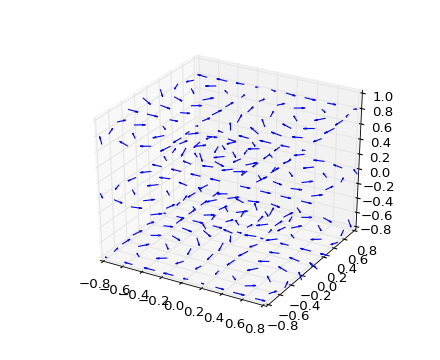## 2D plots in 3D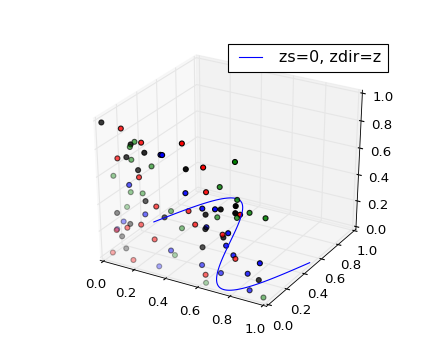## Text

• Axes3D.text(x, y, z, s, zdir=None, **kwargs)

• Add text to the plot. kwargs will be passed on to Axes.text, except for the zdir keyword, which sets the direction to be used as the z direction.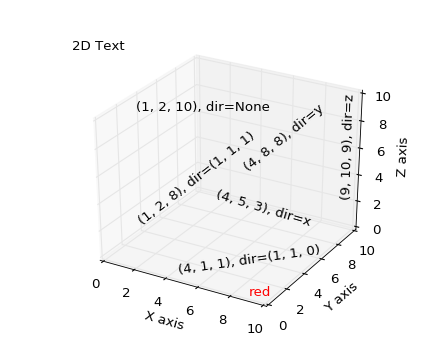## Subplotting

Having multiple 3D plots in a single figure is the same as it is for 2D plots. Also, you can have both 2D and 3D plots in the same figure.

New in version 1.0.0: Subplotting 3D plots was added in v1.0.0.  Earlier version can not do this.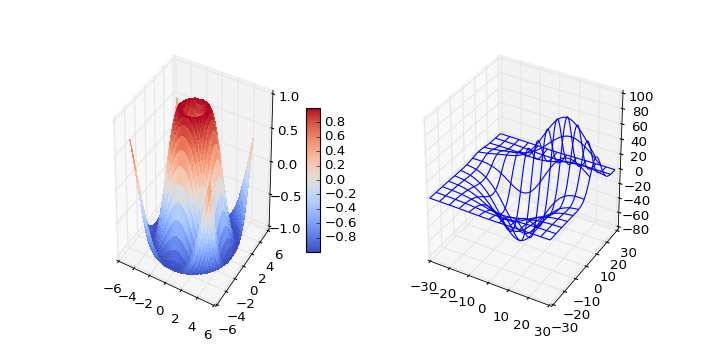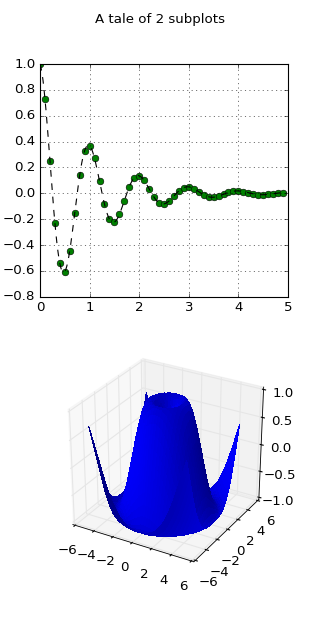### 作者的其它热门文章

1
2 收藏

0 评论
2 收藏
1